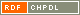Naslov: Distance-balanced graphs: Symmetry conditions Kutnar, Klavdija (Avtor)Malnič, Aleksander (Avtor)Marušič, Dragan (Avtor)Miklavič, Štefko (Avtor)http://dx.doi.org/10.1016/j.disc.2006.03.066 Angleški jezik Delo ni kategorizirano 1.01 - Izvirni znanstveni članek IAM - Inštitut Andrej Marušič A graph ▫$X$▫ is said to be distance-balanced if for any edge ▫$uv$▫ of ▫$X$▫, the number of vertices closer to ▫$u$▫ than to ▫$v$▫ is equal to the number of vertices closer to ▫$v$▫ than to ▫$u$▫. A graph ▫$X$▫ is said to be strongly distance-balanced if for any edge ▫$uv$▫ of ▫$X$▫ and any integer ▫$k$▫, the number of vertices at distance ▫$k$▫ from ▫$u$▫ and at distance ▫$k+1$▫ from ▫$v$▫ is equal to the number of vertices at distance ▫$k+1$▫ from ▫$u$▫ and at distance ▫$k$▫ from ▫$v$▫. Exploring the connection between symmetry properties of graphs and the metric property of being (strongly) distance-balanced is the main theme of this article. That a vertex-transitive graph is necessarily strongly distance-balanced and thus also distance-balanced is an easy observation. With only a slight relaxation of the transitivity condition, the situation changes drastically: there are infinite families of semisymmetric graphs (that is, graphs which are edge-transitive, but not vertex-transitive) which are distance-balanced, but there are also infinite families of semisymmetric graphs which are not distance-balanced. Results on the distance-balanced property in product graphs prove helpful in obtaining these constructions. Finally, a complete classification of strongly distance-balanced graphs is given for the following infinite families of generalized Petersen graphs: GP▫$(n,2)$▫, GP▫$(5k+1,k)$▫, GP▫$(3k 3,k)$▫, and GP▫$(2k+2,k)$▫. graph theory, graph, distance-balanced graphs, vertex-transitive, semysimmetric, generalized Petersen graph 2006 str. 1881-1894 Vol. 306, iss. 16 0012-365X 519.17 17143912073 59Gradivo ni uvrščeno v področja.

Skupna ocena: (0 glasov) Ocenjevanje je dovoljeno samo prijavljenim uporabnikom.Postavite miškin kazalec na naslov za izpis povzetka. Klik na naslov izpiše podrobnosti ali sproži prenos.

## Sekundarni jezik

Jezik: Angleški jezik teorija grafov, razdaljno uravnoteženi grafi, polsimetrični grafi, posplošeni Petersenov graf, tranzitivni grafi

## Komentarji

Dodaj komentar

izpis_zaKomentiranje

Komentarji (0)
 0 - 0 / 0 Ni komentarjev!

Nazaj